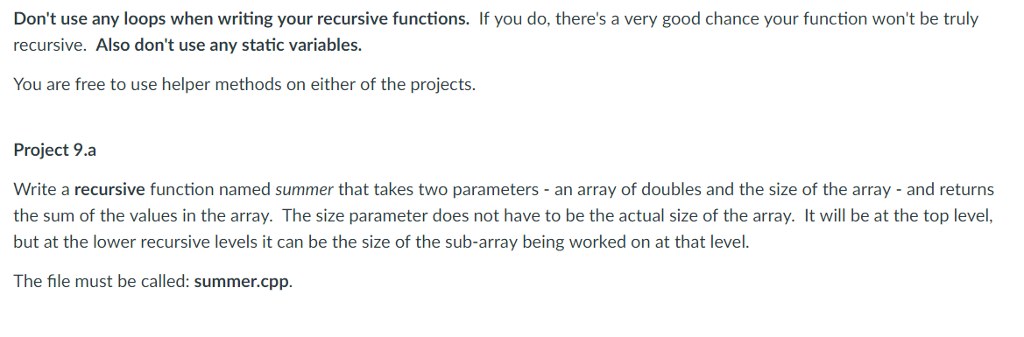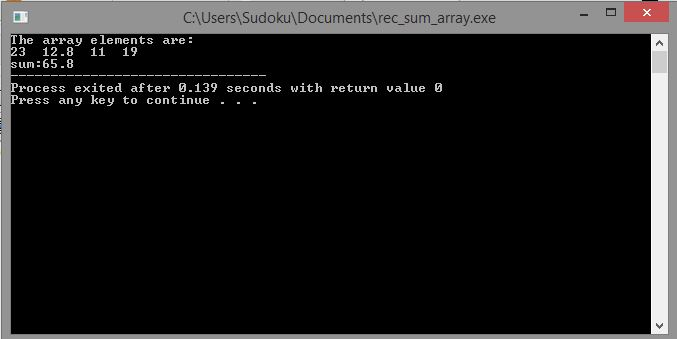# Question & Answer: Don't use any loops when writing your recursive functions. If you do, there's a very good chance your function won't be truly recu…..Don't use plagiarized sources. Get Your Custom Essay on
Question & Answer: Don't use any loops when writing your recursive functions. If you do, there's a very good chance your function won't be truly recu…..
GET AN ESSAY WRITTEN FOR YOU FROM AS LOW AS \$13/PAGE

Don’t use any loops when writing your recursive functions. If you do, there’s a very good chance your function won’t be truly recursive. Also don’t use any static variables. You are free to use helper methods on either of the projects. Write a recursive function named summer that takes two parameters – an array of doubles and the size of the array – and returns the sum of the values in the array. The size parameter does not have to be the actual size of the array. It will be at the top level, but at the lower recursive levels it can be the size of the sub-array being worked on at that level. The file must be called: summer.cpp

#include<iostream>

using namespace std;

//recursive function

double summer(double arr[],int size)

{

//this is the base condition to terminate

//from recursive method

//when size becames 0, then there are no elements to sum up

if(size==0)

return 0;

//if size not 0, then

else

//sum up last indexed element with remaining elements in the array

return arr[size-1]+summer(arr,size-1);

//this will be calculated like this

//1. 19+summer(arr,3)

//2. 19+11+summer(arr,2)

//3. 19+11+12.8+summer(arr,1)

//4. 19+11+12.8+23+summer(arr,0)

//5. base condition

// 19+11+12.8+23+0

}

//main function

int main()

{

//array with double data type

double arr[]={23.0,12.8,11,19};

//displaying array’s elements

cout<<“The array elements are:n”;

for(int i=0;i<4;i++)

cout<<arr[i]<<” “;

cout<<“n”;

//calling summer recursive funtion with

//parameters array and sizeof the array

cout<<“sum:”<<summer(arr,sizeof(arr)/sizeof(arr));

//sizeof(arr)/sizeof(arr) will be size of the array

}